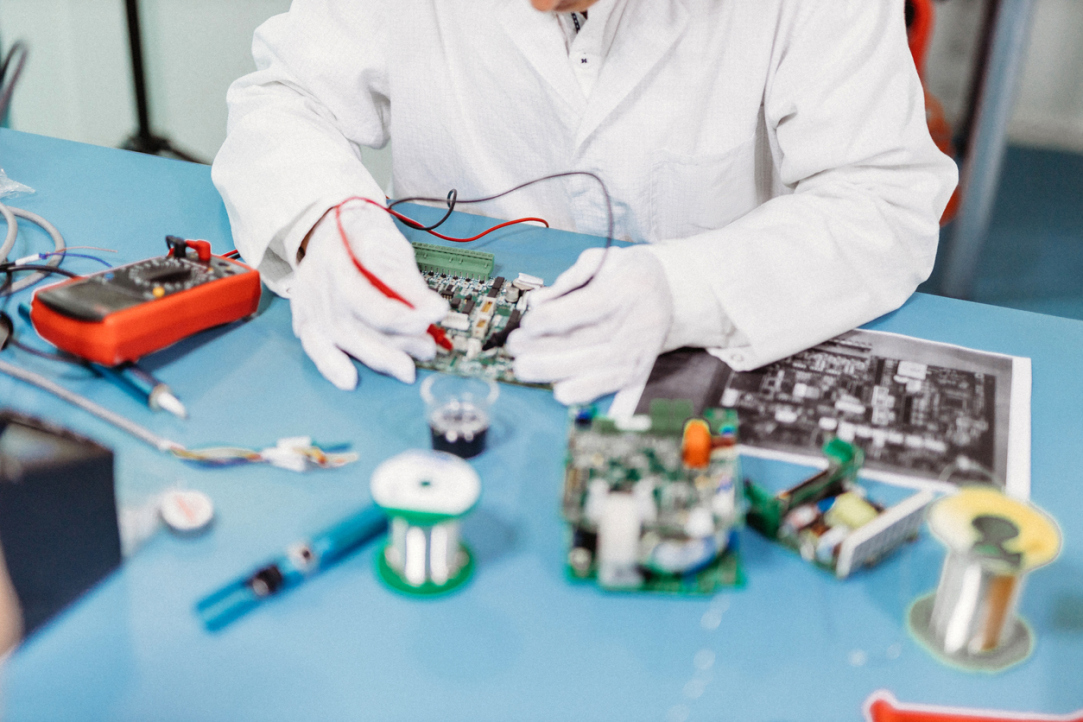• A
• A
• A
• ABC
• ABC
• ABC
• А
• А
• А
• А
• А
Regular version of the site
Campus inMoscow

# Chemists Suggest Using Polymeric Ionic Liquids in SupercapacitorsA team of researchers from HSE MIEM joined colleagues from the Institute of Non-Classical Chemistry in Leipzig to develop a theoretical model of a polymeric ionic liquid on a charged conductive electrode. They used approaches from polymer physics and theoretical electrochemistry to demonstrate the difference in the behaviour of electrical differential capacitance of polymeric and ordinary ionic liquids for the first time. The results of the study were published in Physical Chemistry Chemical Physics.

Polymerised ionic liquids (PIL) are a relatively new class of materials with increasing applications in various fields, from the development of new electrolytes to the creation of solar cells. Unlike ordinary room temperature ionic liquids (liquid organic salts in which cations and anions move freely), in PILs, cations are usually linked in long polymeric chains, while anions move freely. In recent years, PILs have been used (along with ordinary ionic liquids) as a filling in the production of supercapacitors.

Supercapacitors are devices that store energy in an electric double layer on the surface of an electrode (as in electrodes of platinum, gold and carbon, for example). Compared, for example, to an accumulator, supercapacitors accumulate more energy and do so faster. The amount of energy a supercapacitor is able to accumulate is known as its ‘capacitance’.

The authors of the paper were the first to create a theoretical model of the behaviour of capacitance in an electric double layer in PILs and their solutions on the interface with a charged electrode in supercapacitors.

The scholars also compared the PILs’ capacitance with that of ordinary room temperature ionic liquids. The study resulted in a new analytical expression for capacitance under low voltage, which can be used in engineering calculations.

The authors looked at two model cases of interactions between polymerised ions with the surface of the electrode and found both quantitative and qualitative differences in capacitance behaviour from that in ordinary ionic liquids. The researchers predict a huge increase in capacitance for PILs as compared to regular ionic liquids with the same chemical composition.

The study was the first to clarify how the presence of linked cations impacts the electrochemical properties of ionic liquid on a charged electrode.

They say that despite the fact that the developed model is a rather crude description of PILs on charged electrodes, even at this stage of theoretical development, the results can be useful in the development of new supercapacitors, fuel cells, accumulators and solid-state electrolytes.#### Yury Budkov, MIEM HSE Professor

‘We had assumed that the charged polymeric chains in polymeric ionic liquids would attract ions to a charged electrode much easier than in the case of ordinary ionic liquids, where ions are not linked. This, in turn, would have to lead to higher capacitances at the same voltages. After a rigorous calculation based on a combination of approaches from theoretical electrochemistry and polymer physics, we confirmed that our hypothesis was correct. We hope to get experimental proof soon.’